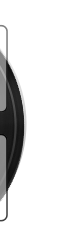3080•听友217997750

^_^^_^^_^^_^^_^^_^^_^太好听 太给力 太喜欢😘^_^^_^^_^^_^^_^^_^^_^^ _ ^